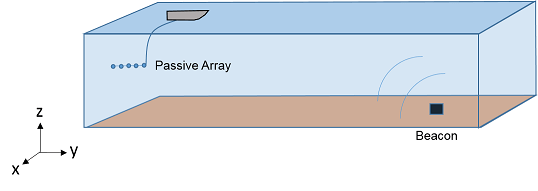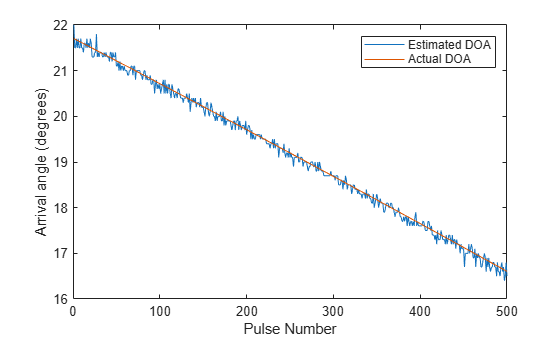# Locating an Acoustic Beacon with a Passive Sonar System

This example shows how to simulate a passive sonar system. A stationary underwater acoustic beacon is detected and localized by a towed passive array in a shallow-water channel. The acoustic beacon transmits a 10 millisecond pulse at 37.5 kilohertz every second, and is modeled as an isotropic projector. The locator system tows a passive array beneath the surface, which is modeled as a uniform linear array. Once the acoustic beacon signal is detected, a direction of arrival estimator is used to locate the beacon.

### Define the Underwater Channel

In this example, the acoustic beacon is located at the bottom of a shallow water channel, which is 200 meters deep. A passive array is towed beneath the surface to locate the beacon.First, create a multipath channel to transmit the signal between the beacon and passive array. Consider ten propagation paths including the direct path and reflections from the top and bottom surfaces. The paths generated by `isopaths` will be used by the multipath channel, `channel`, to simulate the signal propagation.

```propSpeed = 1520; channelDepth = 200; OperatingFrequency = 37.5e3; isopaths = phased.IsoSpeedUnderwaterPaths('ChannelDepth',channelDepth,... 'NumPathsSource','Property','NumPaths',10,'PropagationSpeed',propSpeed); channel = phased.MultipathChannel('OperatingFrequency',OperatingFrequency);```

### Define the Acoustic Beacon and Passive Array

Acoustic Beacon Waveform

Define the waveform emitted by the acoustic beacon. The waveform is a rectangular pulse having a 1 second repetition interval and 10 millisecond width.

```prf = 1; pulseWidth = 10e-3; pulseBandwidth = 1/pulseWidth; fs = 2*pulseBandwidth; wav = phased.RectangularWaveform('PRF',prf,'PulseWidth',pulseWidth,... 'SampleRate',fs); channel.SampleRate = fs;```

Acoustic Beacon

Next, define the acoustic beacon, which is located 1 meter above the bottom of the channel. The acoustic beacon is modeled as an isotropic projector. The acoustic beacon waveform will be radiated to the far field.

```projector = phased.IsotropicProjector('VoltageResponse',120); projRadiator = phased.Radiator('Sensor',projector,... 'PropagationSpeed',propSpeed,'OperatingFrequency',OperatingFrequency); beaconPlat = phased.Platform('InitialPosition',[5000; 2000; -199],... 'Velocity',[0; 0; 0]);```

Passive Towed Array

A passive towed array will detect and localize the source of the pings, and is modeled as a five-element linear array with half-wavelength spacing. The passive array has velocity of 1 m/s in the y-direction. The array axis is oriented parallel to the direction of travel.

```hydrophone = phased.IsotropicHydrophone('VoltageSensitivity',-150); array = phased.ULA('Element',hydrophone,... 'NumElements',5,'ElementSpacing',propSpeed/OperatingFrequency/2,... 'ArrayAxis','y'); arrayCollector = phased.Collector('Sensor',array,... 'PropagationSpeed',propSpeed,'OperatingFrequency',OperatingFrequency); arrayPlat = phased.Platform('InitialPosition',[0; 0; -10],... 'Velocity',[0; 1; 0]);```

Define the receiver amplifier for each hydrophone element. Choose a gain of 20 dB and noise figure of 10 dB.

```rx = phased.ReceiverPreamp(... 'Gain',20,... 'NoiseFigure',10,... 'SampleRate',fs,... 'SeedSource','Property',... 'Seed',2007);```

### Simulate the Passive Sonar System

Activate the acoustic beacon and transmit ten pings. After the propagation delay, the pings appear as peaks in the received signals of the array.

```x = wav(); numTransmits = 10; rxsig = zeros(size(x,1),5,numTransmits); for i = 1:numTransmits % Update array and acoustic beacon positions [pos_tx,vel_tx] = beaconPlat(1/prf); [pos_rx,vel_rx] = arrayPlat(1/prf); % Compute paths between the acoustic beacon and array [paths,dop,aloss,rcvang,srcang] = ... isopaths(pos_tx,pos_rx,vel_tx,vel_rx,1/prf); % Propagate the acoustic beacon waveform tsig = projRadiator(x,srcang); rsig = channel(tsig,paths,dop,aloss); % Collect the propagated signal rsig = arrayCollector(rsig,rcvang); % Store the received pulses rxsig(:,:,i) = abs(rx(rsig)); end```

Plot the last received pulse. Because of the multiple propagation paths, each ping is a superposition of multiple pulses.

```t = (0:length(x)-1)'/fs; plot(t,rxsig(:,end)) xlabel('Time (s)'); ylabel('Signal Amplitude (V)')```### Estimate the Direction of Arrival

Estimate the direction of arrival of the acoustic beacon with respect to the array. Create a MUSIC estimator object, specifying a single source signal and the direction of arrival as an output. Use a scan angle grid with 0.1 degree spacing.

```musicspatialspect = phased.MUSICEstimator('SensorArray',array,... 'PropagationSpeed',propSpeed,'OperatingFrequency',... OperatingFrequency,'ScanAngles',-90:0.1:90,'DOAOutputPort',true,... 'NumSignalsSource','Property','NumSignals',1);```

Next, collect pings for 500 more repetition intervals. Estimate the direction of arrival for each repetition interval, and compare the estimates to the true direction of arrival.

```numTransmits = 500; angPassive = zeros(numTransmits,1); angAct = zeros(numTransmits,1); for i = 1:numTransmits % Update array and acoustic beacon positions [pos_tx,vel_tx] = beaconPlat(1/prf); [pos_rx,vel_rx] = arrayPlat(1/prf); % Compute paths between acoustic beacon and the array [paths,dop,aloss,rcvang,srcang] = ... isopaths(pos_tx,pos_rx,vel_tx,vel_rx,1/prf); angAct(i) = rcvang(1,1); % Propagate the acoustic beacon waveform tsig = projRadiator(x,srcang); rsig = channel(tsig,paths,dop,aloss); % Collect the propagated signal rsig = arrayCollector(rsig,rcvang); rxsig = rx(rsig); % Estimate the direction of arrival [~,angPassive(i)] = musicspatialspect(rxsig); end```

Plot the estimated arrival angles and the true directions of arrival for each pulse repetition interval.

```plot([angPassive angAct]) xlabel('Pulse Number') ylabel('Arrival angle (degrees)') legend('Estimated DOA','Actual DOA')```The estimated and actual directions of arrival agree to within less than one degree.

### Summary

In this example, the transmission of acoustic pings between a beacon and passive array was simulated in a shallow-water channel. Each ping was received along ten acoustic paths. The direction of arrival of the beacon was estimated with respect to the passive array for each received ping and compared to the true direction of arrival. The direction of arrival could be used to locate and recover the beacon.

### Reference

Urick, Robert. Principles of Underwater Sound. Los Altos, California: Peninsula Publishing, 1983.